# Drawing and Interpreting Bar Charts

Drawing and Interpreting Bar Charts• Here is a bar chart showing pupils’ favourite colours.
• The different categories on a bar chart are listed along the bottom.
• These are the different choices available, which in this case are the different colours.
• The frequency, or number in each category goes along the vertical axis (upwards).
• We have the number of pupils on the axis going upwards.
• Each bar is drawn so that the top of each bar is in line with the number in each category.
• 3 pupils choose green so the top of the bar in the green category reaches 3.
• 5 pupils like blue.
• 4 pupils choose red.
• 1 pupil chooses pink.
• 2 pupils choose yellow.
• We give our bar chart a title so that people can understand what it is showing.
• The title for this bar chart is ‘Bar chart to show pupils’ favourite colours.’

The height of each bar shows the number in each category.

Read the number that is in line with the top of each bar.• What is the difference between the number of pupils who chose red and the number of pupils who chose yellow?
• To see how many people each bar represents, read the number on the vertical axis (on the left) that is in line with the top of each bar.
• The top of the red bar is in line with 4.
• 4 pupils prefer red.
• The top of the yellow bar is in line with 2 and so, 2 pupils prefer yellow.
• To find the difference, we subtract.
• 4 – 2 = 2.
• 2 more people prefer red than yellow.Supporting Lessons# Drawing and Interpreting Bar Charts

## Drawing Bar Charts

To draw a bar chart follow these steps:

• Count the number of different categories
• Use a ruler to draw a horizontal axis which is as long enough to record all of the categories.
• Label this axis with the different categories.
• Use a ruler to draw a vertical axis which is as long as the largest number in any of the categories.
• Label this axis with ‘Frequency’ or ‘Number’.
• Look at the number in the each category and draw a bar that is as tall as this number.
• You should leave spaces between the different bars in a bar chart.
• Each bar can be shaded differently or coloured in a different colour.
• A title should be put on the bar chart explaining what is being plotted.

We will draw a bar chart to represent the following data.

The following table shows the favourite colours of pupils in a class. The number below each colour is how many pupils prefer that colour.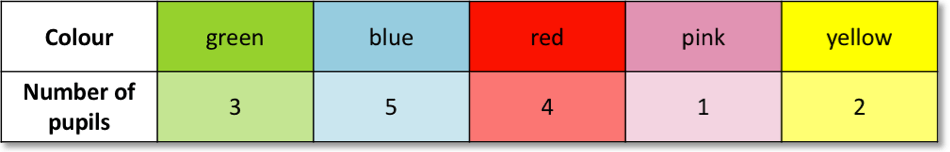We will start by drawing a simple bar chart with no gaps between the bars. The first step is to draw the axes with a ruler.

There are 5 different categories to choose from. Pupils can choose between:

Green, blue, red, pink or yellow.

So our horizontal axis that we draw will be 5 squares along.

The largest number in our table is 5. 5 pupils prefer blue.

So our vertical axis that we draw will also be 5 squares up.The next step is to label the axes.

The categories always go on the horizontal axis, so we write green, blue, red, pink & yellow below each square on the axis. These categories are colours, so our axis title is ‘Colour’.

The vertical axis is frequency, which means number. However if we know what it is that we are counting then we should also write this. We are counting the pupils in the class so our axis title will be ‘Number of pupils’.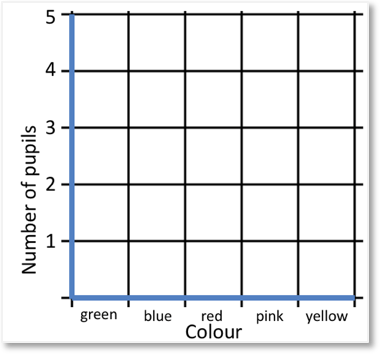We now how our axes ready to plot bars on.

When drawing a bar chart the frequency (or number) always goes on the vertical axis and the categories always go on the horizontal axis.

We now draw the bars on the bar chart.

We look at the number in the first category. We will draw a bar going up in the squares above the green label.

3 pupils like green.

We read up the vertical axis on the left until we see 3. We draw a line across the first square at 3 and shade all of the square below it.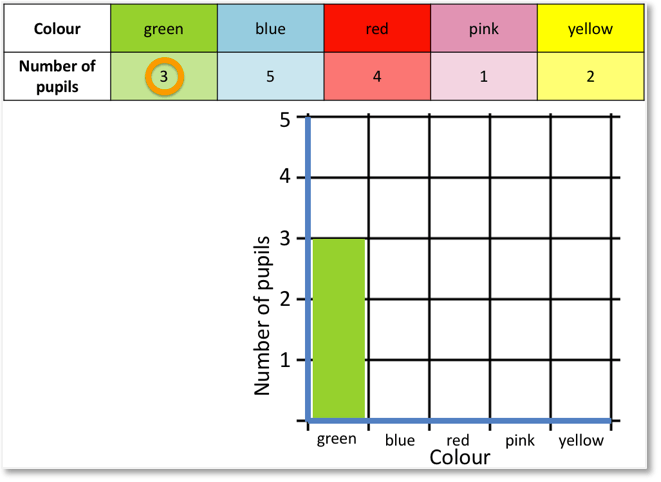The bar is 3 squares high which represents the 3 pupils that like green most.

Different colours can be used for each bar.

Since we are talking about colours, we can use the same colour for each bar as the colour it represents.

5 pupils like blue so we draw a bar that is 5 squares high above the blue category label.

The top of this bar is in line with the 5 on the left axis.

This bar can be shaded in blue because it represents the pupils who like blue.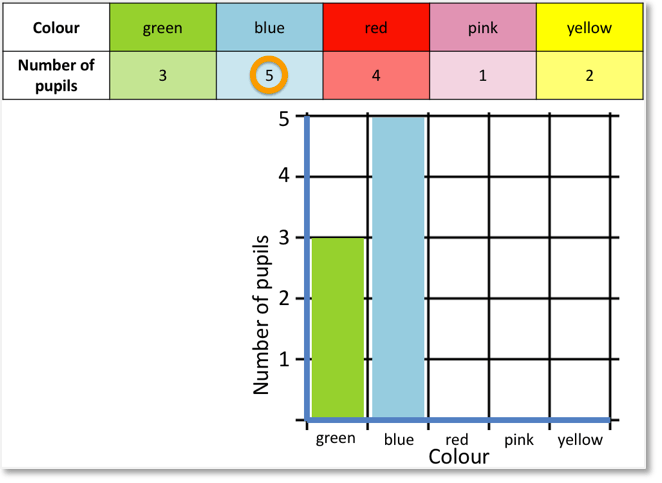4 pupils like red and so a red bar is drawn that is in line with the 4 on the vertical axis.

1 pupil likes pink and 2 pupils like yellow.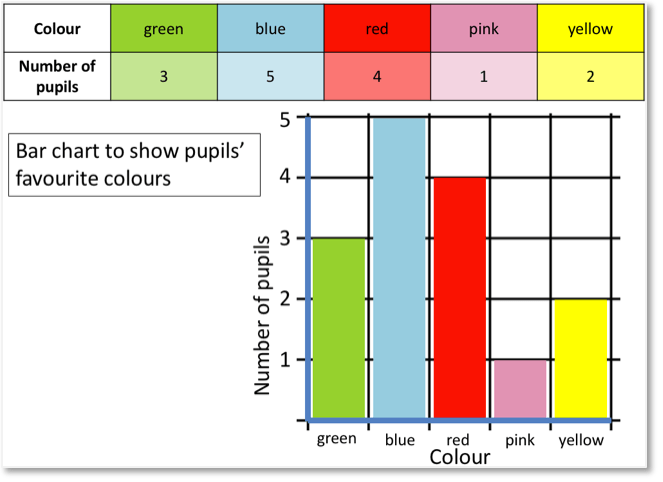Here is the complete bar chart.

The final step is to give the bar chart a title. The title explains what the bar chart is showing.

The title of this bar chart is ‘Bar chart to show pupils’ favourite colours.This was an example of drawing a simple bar chart.

We did not put a space in between the bars.

Bar charts should have a space between the bars when different categories are plotted.

Here is an example of drawing a bar chart to show the method of transport for each pupil in the class.

There are 3 different categories: walk, bus or car.

In this example we put a space between each of the 3 bars to separate them.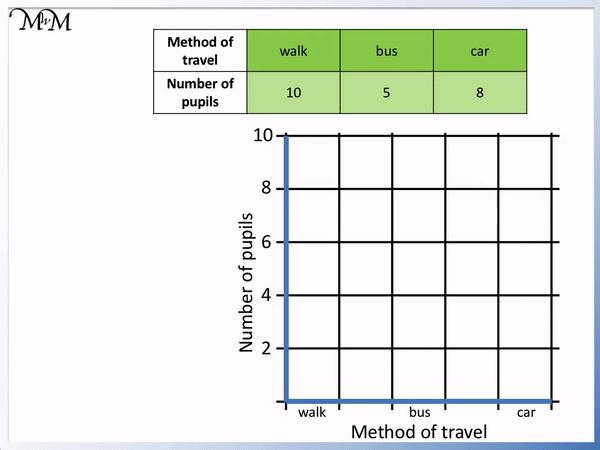10 pupils walk, 5 pupils take the bus and 8 pupils travel by car.

Don’t forget to label the axes and give the bar chart a title.

To read a bar chart read the number on the vertical axis that is in line with the top of each bar. This in the number represented by each bar.

We will look at an example of reading a bar chart showing pupils’ favourite colours.We are asked to find the total number of pupils in the class. This means that we need to find the number of pupils who prefer each colour and then add them up.

To see how many each bar is worth, we read the number that is in line with the top of the bar.3 pupils prefer green, 5 pupils prefer blue, 4 pupils prefer red, 1 pupil prefers pink and 2 pupils prefer yellow.

Alternatively, we can count the number of squares in each bar in this example because each square is worth 1. We might not always be able to count squares if the scale on the axis is different. The easiest way is to read the values from the top of each bar.The total number of pupils in the class is 3 + 5 + 4 + 1 + 2.

3 plus 5 equals 8. Adding 4 more equals 12. Adding 1 equals 13 and 2 more is 14. There are 14 pupils in the class.

Here is another example of reading a bar chart.

Again, we are asked for the total number of pupils in the class on this bar chart.

There are three bars. To read how many people each bar represents, we read the number on the vertical axis that is in line with the top of each bar.

10 people walk to school.

The bar for the bus is exactly in between 4 and 6. The number in between 4 and 6 is 5. So 5 people take the bus to school.

8 people take the car to school.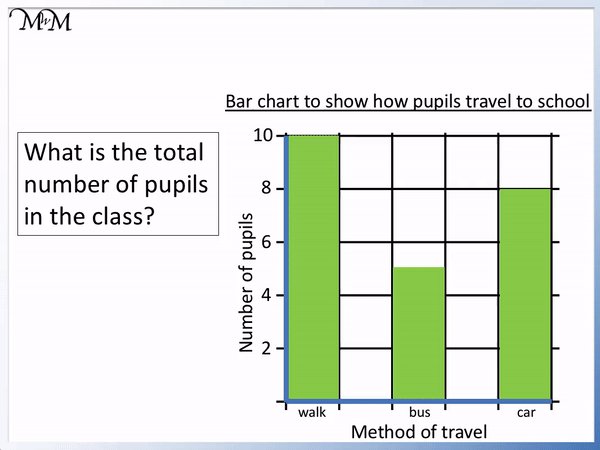We add up these numbers to find the total number in the class.

10 + 5 + 8 = 23 and so, there are 23 people in this class.

Notice in this example that there are 5 people who take the bus to school. We had to read between the numbers 4 and 6 to read this. This is because each square is worth 2 people.

We need to be careful to read from the top of each bar. A ruler can help us to read bar charts as we can line the ruler up with the top of the bar and use it to see which number it is in line with.

## Interpreting Bar Charts

Here are some examples of interpreting bar charts.

In this example we are asked, “What is the most popular colour?”The most popular colour is the colour that is picked by the most pupils.

This means that we want the bar that represents the biggest number. To find this easily, we can just look for the tallest bar.

The tallest bar is the blue bar. 5 pupils prefer blue and so, blue is the most popular colour.The next question asks us, “What is the least popular colour?”The least popular colour is the colour that is picked by the fewest number of pupils.

The least popular colour is the bar that represents the smallest number of pupils.

We can easily find this because it will be the shortest bar on the bar chart.The shortest bar is the pink bar. It is only 1 square high and represents 1 pupil.

Pink is the least popular colour because it is the shortest bar.

The next question asks us for the difference between the most popular and the least popular colours.The most popular colour was blue with 5 pupils.

The least popular colour was pink with only 1 pupil.In maths, when we use the word difference, we are often talking about a subtraction.

5 – 1 = 4

4 more people prefer blue than pink.Here is another example of finding the difference between values on a bar chart.

We are asked for the difference between the number of pupils who chose red and the number of pupils who chose yellow.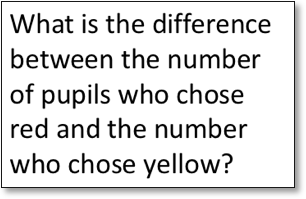4 pupils chose red and 2 pupils chose yellow.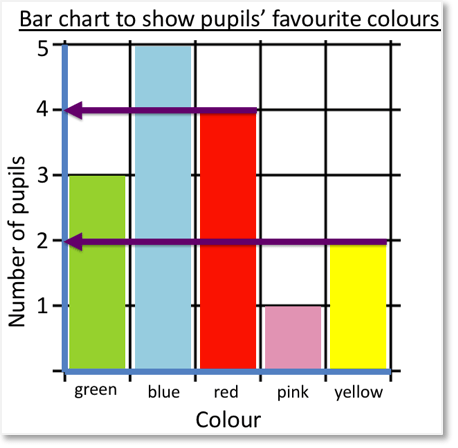To find the difference between two values we subtract them.

4 – 2 = 2

And so, 2 more people prefer red than the number of pupils who prefer yellow.In these next examples of interpreting bar charts we will look at the bar chart showing how pupils travel to school.

We are asked, “What is the most common method of travel?”

The most common method is the method that has the most number of people using it.We want the bar that represents the most number of people. This is the tallest bar.

The tallest bar is for walking. 10 people walk to school and this is the most common method of travel.

This next example of interpreting bar charts asks us for the least common method of travel.

This time we want the bar that represents the fewest number of people.

We want the shortest bar.The shortest bar is the bar for travelling by bus.

The top of this bar is between 4 and 6. 5 people take the bus.

In this question we are asked, “How many more pupils walk than travel by car?”

To find how many more, we need to subtract.

10 pupils walk.

8 pupils travel by car.10 – 8 = 2

So 2 more people walk than travel by car.

We can see that each square is worth 2 people and the bar for walking is one square higher than the bar for travelling by car.

In this example we are asked, “How many more pupils walk than travel by bus?”

Again this question is asking us to find the difference so we will be subtracting.

10 pupils walk and only 5 travel by bus.10 – 5 = 5

And so, 5 more people walk than travel by bus.

We know that 5 is half of 10 and we can see that the bus bar is half the height of the walking bar.Now try our lesson on Finding the Range where we learn what the range is and how to calculate it.